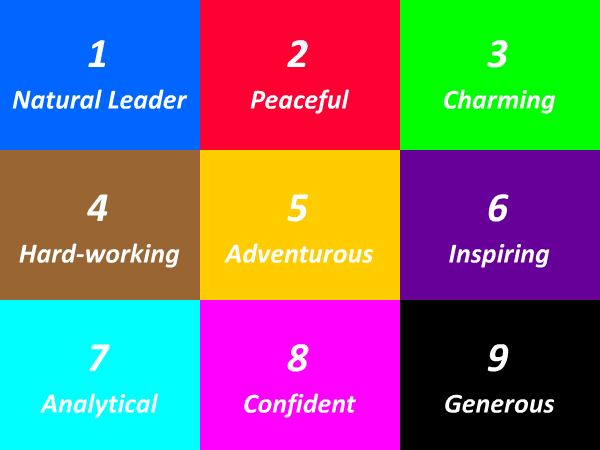# 99 Times Table - Generate Multiplication Table of 99

99 Times Table
1x99=99
2x99=198
3x99=297
4x99=396
5x99=495
6x99=594
7x99=693
8x99=792
9x99=891
10x99=990
11x99=1089
12x99=1188
13x99=1287
14x99=1386
15x99=1485
16x99=1584
17x99=1683
18x99=1782
19x99=1881
20x99=1980
21x99=2079
22x99=2178
23x99=2277
24x99=2376
25x99=2475
99 Times Table
26x99=2574
27x99=2673
28x99=2772
29x99=2871
30x99=2970
31x99=3069
32x99=3168
33x99=3267
34x99=3366
35x99=3465
36x99=3564
37x99=3663
38x99=3762
39x99=3861
40x99=3960
41x99=4059
42x99=4158
43x99=4257
44x99=4356
45x99=4455
46x99=4554
47x99=4653
48x99=4752
49x99=4851
50x99=4950
99 Times Table
51x99=5049
52x99=5148
53x99=5247
54x99=5346
55x99=5445
56x99=5544
57x99=5643
58x99=5742
59x99=5841
60x99=5940
61x99=6039
62x99=6138
63x99=6237
64x99=6336
65x99=6435
66x99=6534
67x99=6633
68x99=6732
69x99=6831
70x99=6930
71x99=7029
72x99=7128
73x99=7227
74x99=7326
75x99=7425

## Test Your Skills Here

The 95 times table quiz is very useful in the improvement of kid's multiplication and maths skills. Here you will find a selection of times table tests designed to help your child to learn and practice their times tables. Fill in your answers. Once you have entered all the answers, click on 'Check' button to see whether you have got them all correct. If your answers is Wrong then we will show you the 'Correct' answer.

Practice Excersise
95 x 25 =
95 x = 95
95 x = 2660
95 x 26 =
95 x = 760
95 x = 570
95 x 30 =
95 x = 950
95 x 23 =
95 x 27 =#### More Tables#### Top Search Quesries for this page:

• 95 times table
• 95 times tables
• table of 95
• 95 multiplication table
• multiplication chart 95
• multiplication of 95
• 95 table maths### Spreading Knowledge Across the World

USA - United States of America  Canada  United Kingdom  Australia  New Zealand  South America  Brazil  Portugal  Netherland  South Africa  Ethiopia  Zambia  Singapore  Malaysia  India  China  UAE - Saudi Arabia  Qatar  Oman  Kuwait  Bahrain  Dubai  Israil  England  Scotland  Norway  Ireland  Denmark  France  Spain  Poland  and  many more....